Next: Cyclical Software Engineering Methodology Up: Supporting Theorems Previous: Supporting Theorems

## Sequential Software Engineering Methodology

In this section, a family of theorems are presented that pertain to the sequential software engineering methodology. The sequential software engineering methodology will find solutions in static environments but not in dynamic environments. If a solution exists, and the sequential software engineering methodology finds the solution, the best case performance is O(N), the worst case performance is O(N), while the average case performance is O(N) where N is the total number of steps in the environment.

Theorem 2 (Sequential: Static Complete)   A sequential software engineering methodology is static complete.

Let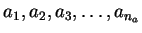be the na requirements leading to a possible analysis A with a1 the initial problem statement. Let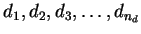be the nd architecture elements leading to a possible design D. Let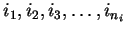be the ni implementation components leading to a possible implementation I. Let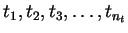be the nt testing suites leading to a possible testing T with tnt being the final system acceptance test. Define a static environment that consists of the four planes of analysis, design, implementation, and testing with each lower plane being a refinement of the higher planes. Define the solution as the tree, or in a more general sense, the directed acyclic graph, of all steps from all four planes used in the final system. This solution can be represented as a sequence of steps <A,D,I,T>.

See Figure 5.5 on pagefor a visual representation of the static environment. See Figure 5.9, Figure 5.10, and Figure 5.11, on pagesthroughfor a visual guide of the reasoning. The numbers associated with the steps represent the order visited by the methodology.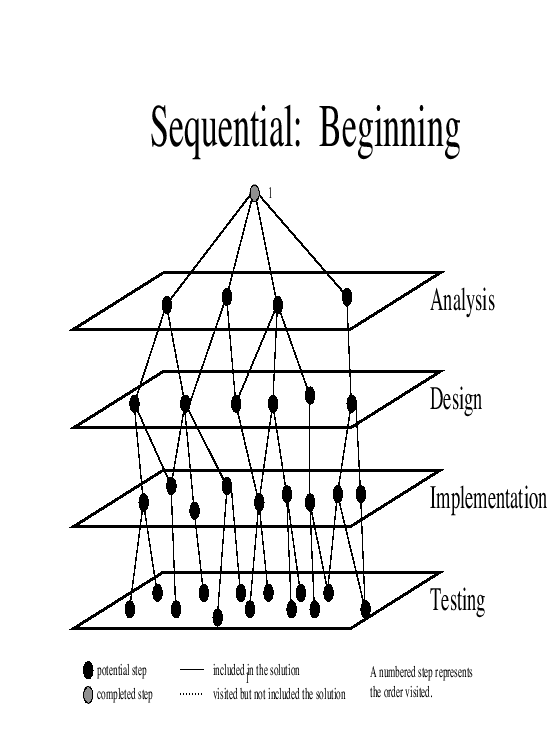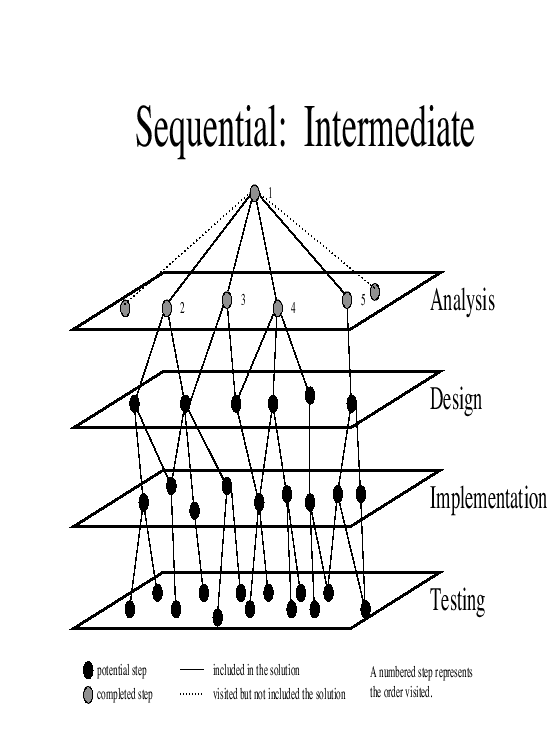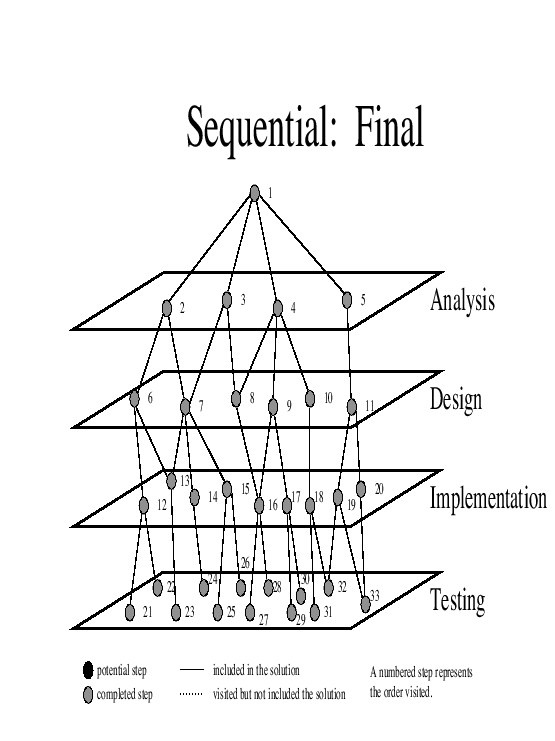Proof 2.1

A sequential software engineering methodology leads to a sequence of steps.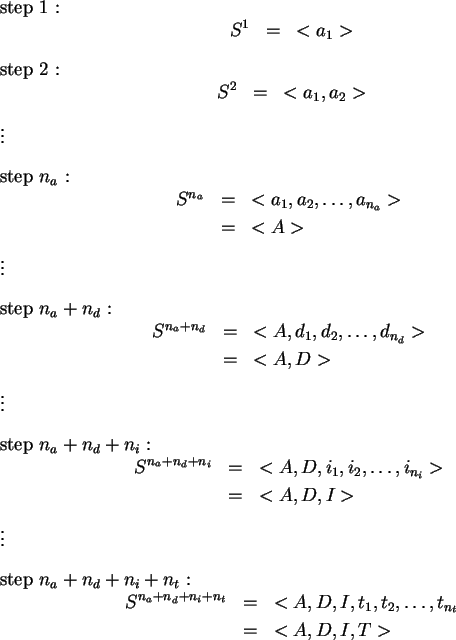The sequence represented by <A,D,I,T> is the solution.

These steps find a solution where steps at the higher levels are exhausted first before going into lower levels. If the environment is static, then all steps in the environment are known before the sequential methodology begins. The sequential methodology first discovers all steps in the analysis plane, followed by all steps in the design plane, followed by all steps in the implementation plane, and then followed by all steps in the testing plane. This can be accomplished because the environment is static and finite. Eventually in the static environment the solution is discovered. Thus, the sequential software engineering methodology is static complete.

Corollary 2.1 (Sequential: Best Case)   The best case performance of the sequential software engineering methodology is O(N).

Proof 2.1.1   Let the environment consist of na analysis steps, nd design steps, ni implementation steps, and nt testing steps. Let the system acceptance test be the very fist step in the testing plane t1. The number of steps to find a solution is na + nd + ni + 1 which is O(N).

Corollary 2.2 (Sequential: Worst Case)   The worst case performance of the sequential software engineering methodology is O(N) where N is the size of the space.

Proof 2.2.1   Let the environment consist of na analysis steps, nd design steps, ni implementation steps, and nt testing steps. Let the system acceptance test be the very last step tn<<1790>>t. The number of steps to find a solution is na + nd + ni + nt which is O(N).

Corollary 2.3 (Sequential: Average Case)   The average case performance of the sequential software engineering methodology is O(N) where N is the size of the space.

Proof 2.3.1   Let the environment consist of na analysis steps, nd design steps, ni implementation steps, and nt testing steps. Let the system acceptance test be the (nt)/2 step in the testing plane t(nt)/2. The number of steps to find a solution is na + nd + ni + (nt)/2 which is O(N).

Corollary 2.4 (Sequential: Dynamic Incomplete)   The sequential software engineering methodology may not find a solution in a dynamic environment.

In a sequential software engineering methodology, all of the high level steps are exercised before proceeding to the next plane of the space. If a new step is introduced once the sequential software engineering methodology has finished with this plane of the environment, the new step will not be part of the solution. The sequential methodology will no longer be able to find the solution.

Let the initial environment consist of na analysis steps, nd design steps, ni implementation steps, and nt testing steps.

Proof 2.4.1

At step na + 1 the sequential software engineering methodology has accomplished the steps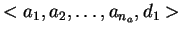. At this time introduce a new requirement an<<1807>>a+1. The sequential software engineering methodology has already finished with the analysis plane and will not discover this new requirement. Thus, a sequential software engineering methodology is dynamic incomplete.

Corollary 2.5 (Sequential: Non-monotonic Incomplete)   The sequential software engineering methodology may not find a solution in a non-monotonic environment.

Proof 2.5.1   The sequential software engineering methodology is incomplete in dynamic environment and thus incomplete to dynamic environments with the non-monotonic property.Next: Cyclical Software Engineering Methodology Up: Supporting Theorems Previous: Supporting Theorems
Ronald LeRoi Burback
1998-12-14